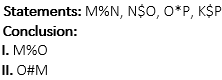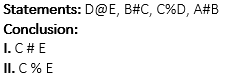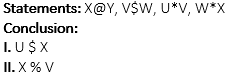We are providing you with the test of the day which will help you in upcoming exams like IDBI Executive 2018. From this, you can practice questions with the timer that will help you to improve speed. IDBI Executive Exam 2018 is one of the biggest opportunity for you guys to grab on, set you own mark and proceed with full confidence. All the very best for your preparations and All the future exams.

Q1. The sum of money is to be divided amongst A, B and C in the respective ratio of 3 : 4 : 5 and another sum of money to be divided between E and F equally. If F got Rs. 1050 less than A, how much amount did B receive?
Rs. 750
Rs. 2000
Rs. 1500
Cannot be determined
None of these

Q2. Three friends Anita, Bindu and Champa divided Rs. 1105 among themselves in such a way that if Rs. 10, Rs. 20 and Rs. 15 are removed from the sum that Anita, Bindu and Champa received respectively, the share of the sum that they got will be in the ratio of 11 : 18 : 24. How much did Champa received ?
Rs. 495
Rs. 510
Rs. 480
Rs. 375
Rs. 445

Q3. On his deathbed, Mr. Kalu called upon his three sons and told them to distribute all his assets worth Rs. 525,000 in the ratio ofamongst themselves. Find the biggest share amongst the three portions.
Rs. 17,5000
Rs. 24,5000
Rs. 10,5000
Rs. 13,2500
Rs. 12,3500

Q4. King Sheru has ordered the distribution of apples according to the following plan : for every 20 apples the elephant gets, the zebra should get 13 apples and the deer should get 8 apples. Now his servant Shambha jackal is in a fix. Can you help him by telling how much should he give to the elephant if there were 820 apples in total?
140
160
200
400
450
Solution:
Ratio of apples obtained by elephant, zebra and deer respectively = 20 : 13 : 8Q5. Two horses started simultaneously towards each other and meet each other 3 hr 20 min later. The ratio of speed of faster horse to that of slower horse is 3 : 1.How much time will it take the slower horse to cover the whole distance if the slower arrived at the place of departure of the faster 5 hours later than the faster arrived at the point of departure of the slower after they meet ?
10 hours
40/3 hours
44/3 hours
16 hours
46/3 hours

Directions (6-10): In the following questions, the symbols @, #, %, \$ and * are used with the following meaning as illustrated below.
‘A @ B’ means ‘A is not smaller than B’
‘A # B’ means ‘A is neither smaller than nor equal to B’
‘A % B’ means ‘A is neither smaller than nor greater than B’
‘A \$ B’ means ‘A is not greater than B’
‘A * B’ means ‘A is neither greater than nor equal to B’

Q6.If only conclusion I is true
If only conclusion II is true
If either conclusion I or II is true
If neither conclusion I nor II is true
If both conclusions I and II are true
Solution:
I. M%O (Not True)
II. O#M (Not True)

Q7.If only conclusion I is true
If only conclusion II is true
If either conclusion I or II is true
If neither conclusion I nor II is true
If both conclusions I and II are true
Solution:
I. A # E (True)
II. D @ A (Not True)

Q8.If only conclusion I is true
If only conclusion II is true
If either conclusion I or II is true
If neither conclusion I nor II is true
If both conclusions I and II are true
Solution:
I. C # E (Not True)
II. C % E (Not True)

Q9.If only conclusion I is true
If only conclusion II is true
If either conclusion I or II is true
If neither conclusion I nor II is true
If both conclusions I and II are true
Solution:
I. U \$ X (Not True)
II. X % V (Not True)

Q10.If only conclusion I is true
If only conclusion II is true
If either conclusion I or II is true
If neither conclusion I nor II is true
If both conclusions I and II are true
Solution:
I. M @ P (Not True)
II. O # L (True)

Directions (11-15): Read each sentence to find out whether there is any grammatical error or idiomatic error in it. The error, if any, will be in one part of the sentence. The number of that part is the answer. If there is no error, the answer is (5). (Ignore errors of punctuation, if any)

Q11. Non-communicable diseases (1)/ often require (2)/ long term and expensive interventions which (3)/ poor people cannot afford. (4) No error (5).
1
2
3
4
5
Solution:
No Error

Q12. A leader is a group member which (1)/ exerts profound influence (2)/ on the behavior and attitudes (3)/ of other members of the group. (4) No error (5)
1
2
3
4
5
Solution:
Here leader is an animated being. Hence, A leader is a group member who — should be used.

Q13. One of the foremost (1)/ challenges face (2)/ the company is to (3)/ attract and retain talent (4) No error (5).
1
2
3
4
5
Solution:
Here, challenges faced by — should be used.

Q14. Our country can contribute (1)/ to the mitigation of global warming (2)/ by protecting forests (3)/ and starting at afforestation programme. (4) No error (5)
1
2
3
4
5
Solution:
There is no need to use ‘to’ here.

Q15. Santosh succeed (1)/ due to the encouragement (2)/ of friends, relatives (3)/ and well-wishers. (4) No error (5)
1
2
3
4
5
Solution:
Here, Simple Past i.e. Santosh succeeded — should be used.

You May Also Like to Read: